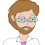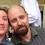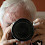## Pic## Friday, November 9, 2012

### How not to teach it: division by zero

What doesn't work

We all agree that this is unsatisfying:
You aren't allowed to divide by zero, because it's a rule.
And many of us (read: me, yesterday) think that this is better:
What's 10 divided by 2? It's 5, because 10 split up evenly into two groups has 5 in each. 10 divided by 1? 10 in 1 group has 10. But 10 in 0 groups? What would that even mean?
And, then, having clearly and elegantly explained why dividing by zero would be a very, very silly thing to do, we go back to the day's main topic:
So 2 to the negative 3rd power is 1/8...
Wait, hold on.

I get it: if you think of division as evenly grouping items, then dividing by zero makes no sense. But that's just the normal wear and tear of a mathematical model. We ask kids to believe that exponentiation is like repeated multiplication, and then we ask them to forget that when we introduce negative powers. Multiplication is repeated addition until you throw in "2.3 times 5.1" and then everything goes to hell. We freaking give quadratics imaginary solutions, and our failure to imagine what "0 groups" looks like is stopping us from dividing by zero? Yeah, right.

(Also, wouldn't zero groups have no items in them?)

And another thing: we tell kids that division by zero is undefined. Skeptically, they take out their calculators and punch some keys and get an error message. "Wait! He's right. It gives you an error."

When it comes to dividing by zero, there is a lot wrong with the standard teachery maneuvers:

• Any sort of "wtf would 0 groups mean" argument does not show that division by 0 is non-sensible. All that it shows is that this particular model of division -- the grouping model -- breaks down for non-integers. That's normal in math. Kids should be regularly creating and discarding conceptual models.
• "Undefined" is the best we can do? Language matters, and saying that 5/0 is undefined makes it sound like, shoot, well, we were going to get around to it but we just chose to let it slide.
• The kids are checking their calculators to see if division by zero makes sense. For crying out loud, that's not math. They're wondering whether to believe you or not, because what you're saying doesn't make sense. Hell, everyone knows that 5 divided by 0 is 0. It just makes so much sense...
This works better

March in front of the classroom. "What's 3 divided by 0? Someone tell me NOW," you say.

If your students are a bunch of sissies and nerds they'll shout "You can't divide by zero!"

"Oh don't give me that math teacher stuff. Who says that I can't divide by zero? Give me a real answer."

That's all it takes. Really. They've been waiting in every math class since they were 8 to get this off their chests.

"3 divided by 0 is 0."

OK, cool. Now we've got something to work with. Ask the class, agree or disagree?

But if 3 divided by 0 is 0, and 5 divided by 0 is 0, then wouldn't this follow?
So 5 = 3, right?

They squirm. They try something else. Maybe 5 divided by 0 is 5? You can handle that too. The point is to lead them to contradiction, and let them grapple with that tension. There are other ways to tug out the contradictions.

Here's why this is better:
• We shouldn't be telling kids that the reason that we don't divide by zero is because an intuitively pleasing model fails. That should never stop a good mathematician.
• Telling kids that division by zero is "undefined" sounds lazy. It's more accurate and informative to say that division by zero leads to contradiction.
• How do you help kids see that it leads to contradiction? Take suggestions from the kids of what division by zero should mean, and then let them see the implications. Let them try to make things consistent. Make the choices clear. Sure, we can think of division that way. We'd just have to refine our rule for multiplying fractions. So, what's your new rule for multiplying fractions?
All of this is more authentic than what I used to do. ("What I used to do"? I'm talking about yesterday. Things move fast around here.) The biggest change in what I'm doing this year is slowing down and having kids make arguments in class. Proof and argument is how I'm helping my kids make sense of this stuff. There's no shortcut or substitute.

1.I tend to take the following approach on dividing by zero. "What's 6 divided by 2?" "3" they all yell. "Why?" "Because if you split 6 into 2 groups each will have 3" I take 6 students and split them into a group of 5 and 1. "No. They need to be equal groups," they complain. So I admit that is one way to think about dividing by a positive integer, but it breaks down if we tried to divide something into -3 groups or one-half groups, or pi groups. At this point (or earlier) someone will try to claim there are rules about dividing by negatives or fractions. I say that I don't care about rules...I need understanding. So I want a more general answer as to why 6 divided by 2 is 3, and keep prodding and asking until I get that 3 times 2 is 6. That's how I want them to think about division. So then I ask what is 0 divided by 5. This throws a few students but enough students convince the class that it is 0 because 0 times 5 is 0. Then I ask what 5 divided by 0 is. It's undefined. Why? Because there is no number that multiplies by 0 to get 5. Finally I ask what's 0 divided by 0. That starts an interesting discussion.

1.A different understanding of division is through addition.

'How many times can you add the bottom number to get the top?"

10 / 5 is 2 because to get 10 you can add 5 twice.

12 / 5 is 2.4 because to get 12 you can add 5 twice and then add a .4th of 5. ect ect

So what's 0 / 0? It is 0, or 1 , or 2 , or infinity, or whatever.
You can add 0 however many times you like, ranging into infinity.

Meaning its indefinite or without having a single correct answer.

AKA division by 0 is fine as long as it's by 0, and then it could be any number. (though i know all mathy people absolutely hate when there isn't a single correct answer, but that does NOT mean there IS NO answer)

2.In physics we say that anything divided by zero is infinite. What is usually implied there is that we are taking the limit as the denominator approaches zero. So let's say, for example, that we are talking about Newton's Law of Gravitation and I'm trying to emphasize how the gravitational force is related to the distance between the objects. I say "if the objects are really really really far away from each other, what is the force between them?" Students: "Really small." Me: "As the objects get closer and closer together and the distance between them approaches zero, what is the force between them?" Students: "Undefined."
As you said, "undefined" is a largely meaningless factoid, and in this case it is getting in the way of understanding the relationship between two physical quantities. So I guess the whole point of this is, have you considered having the infinity discussion?

3.Except is it infinity or negative infinity? There's problems with that model, as a Numberphile video pointed out: http://www.youtube.com/watch?v=BRRolKTlF6Q
I particularly like the remarks about 0^0, which seems to work... as long as we restrict ourselves to the basic, boring Cartesian plane.

The model I tend to use is the grouping one, incidentally... 0 divided by 5 means sharing 0 things among five people, so everyone gets zero items. 5 divided by 0 means sharing 5 things among zero people... so I have five items... no one's allowed to get them... and we can't stop here, I'm a person... so I give them to... no, can't do that... er... I suppose I could put them on the desk and inch away, but someone might pick them up later... uh... I throw them into this convenient black hole. There.

4.It is my experience that we use models which are appropriate for our students. Your explanation works great for students who have significant understanding of the equivalence nature of different lines of algebra, but will be just as much hocus-pocus for students who don't get algebra.

I think we should move between models, and expand upon them as needed. We should probably show students a variety of explanations, some of them based on intuition, and others based on formal logic, since both are valuable ways of knowing in mathematics (even though our intuition is sometimes wrong in mathematics, it can lead to some powerful results when it is correct).

5.By the way, I completely agree on you on the "what doesn't work." I've seen that problem so many times...

6.Lovely. All of it lovely.

I only have one thing to add to the conversation. Not to replace or better anything that's been said. Just something that hasn't been said yet.

Don't neglect our friend quotative division, though. When the partitive division (how many would be in each of zero groups?) gives an unsatisfying answer, we can call our quotative friend. To wit: "6 divided by 2 is 3 because if I have 6 things, I can make 3 groups of 2." Here the quotient is the number of groups and the divisor is the size of the group.

So for division by zero, we are asking "If I have 6 cookies, and I am putting zero cookies in each box, how many boxes can I fill?"

This interpretation also makes important contributions to the study of fraction division.

7.I seem to be having serious issues posting here today... I think my morning failure was due to my haste in getting out the door to school, but earlier my browser reset everything again here mid sentence. Weird. Most of what I have to say though I remarked on over in the parallel 'math mistakes' post... in summary, I don't think we should throw out a model just because it only applies to some subset of numbers (like positive ones).

Two additional things: If you're saying 5/0=3/0 implies 5=3, why can't 0/3=0/5=0 imply the EXACT same thing? Yet for some reason we accept that 3 and 5 are different, and division with 0 on top is fine. And second additional thing is just to point at this Numberphile Video that addresses a number of issues of zero for anyone wanting to see their arguments:

1.0/x = 0/5 does not imply that x = 5, though. Why? Because, hiding in the background, is the acceptability of multiplication by zero and the unacceptability of division by zero.

8.I like trying to think of it in terms of quotative and partitive division. I like thinking about this in terms of consistency being something that mathematicians value. (Also nice when discussing 0^0... 1 or 0?)

I'll link my activity for rational functions where we discuss it. It doesn't do anything innovative or novel. I like this issue as a motivator for the shift from numerical to functional thinking. https://docs.google.com/open?id=0B8frwh-y1pyQVUpIRVVUMXh2dmc

9."Proof and argument is how I'm helping my kids make sense of this stuff." So true, and yet proof and argument will also lead your kids into further confusion. Confusion is healthy, if it comes with later resolution. For instance, the classic

$latex 1 = \sqrt{1 \cdot 1} = \sqrt{(-1) \cdot (-1)} = \sqrt{-1} \cdot \sqrt{-1} = -1$

seems to produce an immediate contradiction from admitting the existence of complex numbers. It is a rare student who understands the flaw in this argument while not doubting the logic that lead you from division by 0 to contradiction.

10.I appreciate so much if teachers think about their profession. This is still a rare thing.

The comment of Galois nails down what the university teaches: division is the inverse of multiplication. It is however a rather grown up way to think about operations on sets of numbers. Teachers tell me that this way of thinking is not open for kids until some point in their development and education. Remember the problems the mathematicians had in old times with imaginary numbers, or even with negative numbers!

There are three ways to do math, and correspondingly three areas of our brain: by geometry, by algebra and by logic. E.g., numbers have a geometrical meaning on the number line. Even negative numbers can be found there. (For imaginary numbers, we need the plain.) Numbers can also be used in algebraic manipulations. And logic comes in if we try to prove that the solution we find by any of this means is correct or unique.

As an example, we can prove 12*47=47*12 the geometric way by arranging dots in a square pattern. From that we derive algebraic rules. This does not help us to see if a*x=c determines x in a unique way.

Back to the division by 0: Geometrically, there simply is no rectangle of area 5 with side length 0. Algebraically, we get into troubles, because we want 5/0=a to imply 5=0*a, which is always false. And we need still a bit of logic to see, why 0*a must be 0 in algebraic manipulations.

Another problem: Define 0^0. In math, this is defined as 1 by various reasons, which have to do with algebraic rules of the power function, or with Taylor series. But can you teach that geometrically?

11.This is such a great discussion and one I'm going to use. We have this "great" rule in our school about not testing the day before Thxgiving break - so I'll have a test the day Monday before our break.

On that Tuesday, I'll do something that gets these discussions going with my Algebra I students.

I'm wondering what is the best way to start that discussion and get them to buy in. I'm thinking something along the lines of a big production and "Today you are going to prove all your previous math teachers wrong." Should be fun.

12.Nice post. Has me thinking of other ways this comes up.

Other puzzling results

1/4 * 4 = 1
1/3 * 3 = 1
1/1.8 * 1.8 = 1
so 1/0 * 0 = 1 so ...

Or

50 divided by 100 is 0.5;
50 divided by 10 is 5
50 divided by 1 is 50;
50 divided by 0.1 is 500;
50 divided by 0.01 is 5000;
...
50 divided by 0 is ???

Or

Suppose I told you a scale factor of 2.5 was applied to a line segment and I gave you the new length. Could you tell me the orignal length? Suppose a scale factor of 0 was applied to a line segment and I gave you the new length. Could you tell me the original length?

Or

Are these lines steep or not so steep
y = 0x
y = 1x
y = 5/20x
y = 5/3 x
y = 5/1 x
y = 5/.2 x
y = 5/.01 x
y = 5/0 x

1.These are all solid. And I like how you're bringing a lot of stuff together.

13.Situation 1: You arrive at the Fair and find that each ride costs 2 tickets.
There are 6 tickets remaining at the ticket booth.
How many rides can you take?

Situation 2: You arrive at the Fair and find that each ride costs 2 tickets.
There are 0 tickets remaining at the ticket booth.
How many rides can you take?

Situation 3: You arrive at the Fair and find that each ride costs 0 tickets.
There are 6 tickets remaining at the ticket booth.
How many rides can you take?

1.What I love about this scenario is that it gets at the idea that division by zero ought to result in -- if anything -- an infinite number. I wasn't sure how to get kids to see that other than through dividing by numbers that approach zero, but this would do the trick.

At the same time, I don't want my kids saying that 3/0 = infinity. I want them to see the notion of division by zero as deeply problematic.

At the same time, a lot of folks on twitter and mathmistakes have been pushing me to see the "infinity" perspective, and I'm starting to see what you all mean.

14.I always start with the logical approach--if 8 divided by 0 is 0, then 0*0 should be... showing that the inverse doesn't work.

But with stronger kids I go through the infinity perspective, particularly because you can illustrate it nicely with horizontal and vertical slope as the difference between a numerator and denominator of 0.